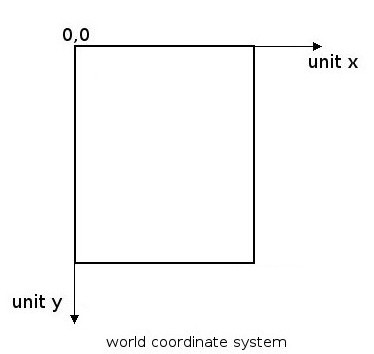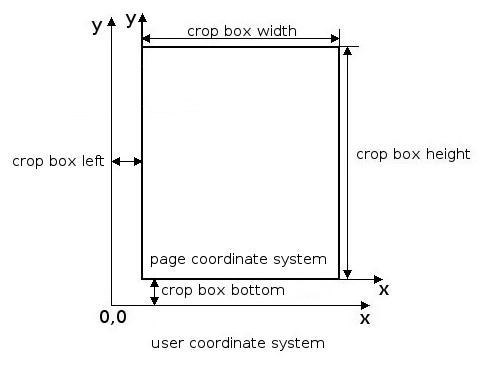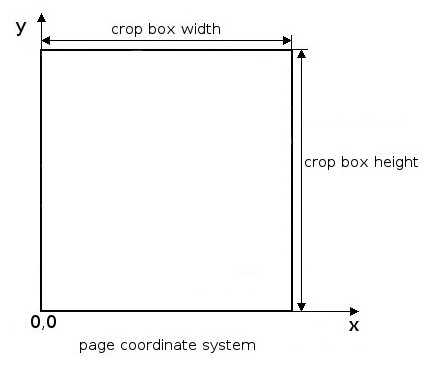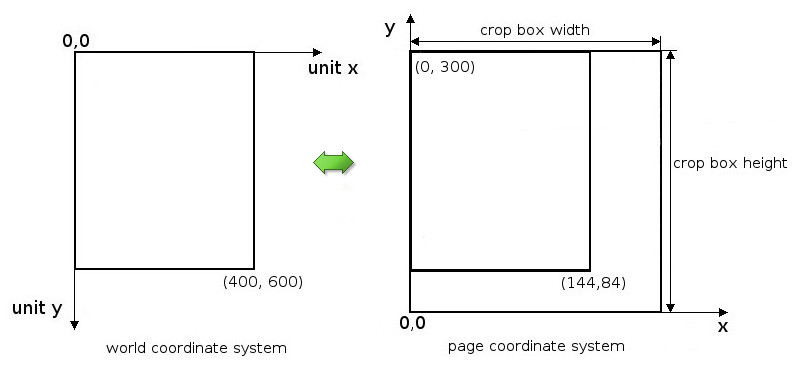.NET Framework 4.5.2+

# Coordinate Systems

The coordinate systems define the position, orientation, and size of text, graphics, and images that appear on a page. The coordinate systems are determined with respect to the current page.

This document describes coordinate systems used in the PDF Document API and how transformations among these coordinate systems are specified.

## World Coordinate System

The world coordinate system models a particular graphics world. The world coordinate system’s origin (0,0) is at the top left of the page. A positive x increases towards the right and a positive y towards the bottom.To learn about API that uses the world coordinate system, see Using Coordinate Systems in API

## User Coordinate system

The positive x axis extends horizontally to the right and the positive y axis vertically upward. The page boundaries are defined by the crop box in the user coordinate system. The crop box designates the visible page area that is displayed or printed. To get the page crop box, use the PdfPageTreeObject.CropBox property. The size of the unit in the user space is a point (1/72 of an inch).

The image below shows the conversion from the user coordinate system to the page coordinate system.The user coordinate system is an internal PDF coordinate system. The document processor’s low-level content API (document model) uses the PDF user coordinate system. A PdfDocument object that can be accessed using the PdfDocumentProcessor.Document property represents the document model (see the How to: Replace a Form Field with an Image example). The high-level API refers to the page coordinate system. So, you do not need to use the user coordinate system in most cases.

## Page Coordinate System

The page coordinate system was introduced to simplify the page calculations. The page coordinate system’s origin (0, 0) is shifted by page crop bottom and page crop box left values relative to the user coordinate system’s origin (see the image above). The lower-left corner of the page coincides with the origin of the page coordinate system (0, 0). The Y axis is directed from the bottom of the page to the top.To learn about API that uses the page coordinate system, see Using Coordinates Systems in API

## Use Coordinate Systems in API

The PdfGraphics class (see the PDF Graphics section for details) uses the world coordinate system.

The PdfAnnotation.Rect and PdfPageTreeObject.CropBox properties use the user coordinate system.

The following API uses the page coordinate system:

Product API
PDF Document API PdfDocumentProcessor.FindText
PdfDocumentProcessor.GetImages
PdfDocumentProcessor.GetText
PdfWord class.
WinForms PDF Viewer PdfViewer.FindText
WPF PDF Viewer PdfViewerControl.FindText
PdfViewerControl.GetText
PdfViewerControl.ScrollIntoView

## Coordinate Transformations

You need to convert page coordinates to world coordinates for example, to highlight search results in a document using the PDF Document API (see How to: Highlight Search Results in a Document for more details). The search results are obtained from the overloaded PdfDocumentProcessor.FindText method in the page coordinates. To draw filled rectangles that correspond to the document area containing found text, convert page coordinates to world coordinates since the PdfGraphics.FillRectangle method uses world coordinates.

Unlike the page coordinates to world coordinates transformation, the world coordinates are transformed to page coordinates automatically using the following PDF Document API’s methods:

The user coordinates should be converted to world coordinates, for example, when you need to substitute a text form field with an image (see How to: Replace a Form Field with an Image for more details).

## Convert World Coordinates to Page Coordinates

To convert a graphics unit into a physical value such as inches, we should provide a resolution’s value (dpi - the number of dots (units) per inch). The dpi shows how many units from the world coordinates are placed in an inch on a page. So, to get the value in inches, we should divide units by dpi values (for example, 400 units divided by 200 dpi equals 2 inches). The measurement unit in the page coordinate system is a point (1/72 of an inch).

The world coordinates (units) are converted to page coordinates (points) using the following formula:

x = (unitX / dpiX) * 72;

y = cropBox.Height - (unitY / dpiY) * 72.

Use the PdfPageTreeObject.CropBox property to get the page crop box height.

Example

Two points represent a rectangle in the world coordinates. The first point is (0, 0), the second point is (400, 600) units and the dpi value is 200.

Using the formula above, we get the following result in the page coordinates:

The first point:

Point X1 value = (0 / 200) * 72 = 0

Point Y1 value = 300 - (0 / 200) * 72 = 300 (equal to crop box height)

The second point:

Point X2 value = (400 / 200) * 72 = 144

Point Y2 value = (300 - (600 / 200) * 72) = 84## Convert Page Coordinates to World Coordinates

The page coordinates (points) are converted to world coordinates (units) using the following formula:

unitX= (x / 72) * dpiX;

unitY = ((cropBox.Height - y) / 72) * dpiY.

Example

Let’s take a rectangle represented by two points obtained from the previous example (see the section above). The first point is (0, 300), the second point is (144, 84) and the dpi value is 200.

Using the formula above, we get the following result:

The first point in the world coordinates:

unitX1= (0 / 72) * 200 = 0;

unitY1 = ((300 - 300) / 72) * 200 = 0.

The second point in the world coordinates:

unitX2= (144 / 72) * 200 = 400;

unitY2 = ((300 - 84) / 72) * 200 = 600.

## Convert User Coordinates to World Coordinates

To perform this conversion, we modify the formula that is used to convert page coordinates to world coordinates

UnitX = (Xu / 72) * dpiX;

UnitY = ((cropBox.Height - Yu) / 72) * dpiY;

where Xu and Yu - user coordinates.

Since the page coordinates system origin (0, 0) is shifted by page crop bottom and page crop box left values relative to the user coordinate system’s origin, the page coordinates are converted to user coordinates using the formula below:

Xu = rect.Left – cropBox.Left;

Yu = rect.Bottom – cropBox.Bottom;

When we substitute Xu and Yu into the user coordinates to world coordinates conversion formula, we get the following:

UnitX = ((rect.Left – cropBox.Left) / 72) * dpiX;

UnitY = ((cropBox.Height – rect.Bottom + cropBox.Bottom) / 72 ) * dpiY = ((cropBox.Top – rect.Bottom) / 72 ) * dpiY.

Use the PdfPageTreeObject.CropBox and PdfAnnotation.Rect properties to get the crop box and annotation rectangle values.

See the How to: Replace a Form Field with an Image example for more details.